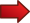# Feet to Miles

Instructions: Use this step-by-step Feet to Miles Calculator, to compute amount of miles corresponding to a given number of feet $$F$$. Please provide the required information in the form below:Number of Feet $$F$$ (number or fraction) =

## More about the conversion of feet to miles

The idea of converting feet to miles may appear to be strange, considering that a foot and a mile are fairly different in sizes, a mile being much bigger.

Typically, there is always a reason for a conversion of magnitude of distances: either we are working with metric systems of different countries, or sometimes just for the pleasure in making things harder (really).

### Feet to Miles conversion

When stating how to do a feet to miles conversion, it is typically easier to start with miles to feet. Indeed, there is a round number for that:

$\large 1 \text{ mile} = 5280 \text{ feet}$

So you need lots of feet to make one mile. Now, taking the above equation and dividing both sides by 5280, we get that

$\large \frac{1}{5280} \text{ mile} = 1 \text{ foot}$

and calculating the numeric form of the fraction we get:

$\large 1 \text{ foot} = 0.000189394 \text{ miles}$So then, you may be asking yourself now: How many feet are in a mile? . The answer is, there are 5280 feet in a mile.And you may ask yourself: " How many miles are there in a foot? ". The answer is that there are 0.000189394 miles in a foot.

Hence, if we have $$F$$ feet, the corresponding amount of miles is

$\large F \text{ feet} = 0.000189394 \times F \text{ miles}$

### So based on the above, how do you convert miles into feet?

The formula above is telling to get the number of feet, and multiply that by 0.000189394, to get the corresponding number of miles.

### Examples

Convert 41 feet into miles

Solution: In this case we get $$F = 41$$ feet, so we can apply directly the formula above to say that

$\large F \text{ feet} = 0.000189394 \times 41 \text{ miles} = 0.007765154 \text{ miles}$

### Other uses of the feet-to-miles conversion

Sometimes, the conversion of feet-to-miles is done as an intermediary step for making a conversion for a velocity unit. For example, we may be interested in converting speed given in feet per second to miles per hour.

We know that 1 mile is equal to 5280 feet. Also, we know that 1 hour is the same as $$60 \times 60 = 3,600$$ seconds. Therefore,

$\large \displaystyle 1 \text{ } \frac{\text{mile}}{\text{hour}} = \frac{5280 \text{ feet}}{3600 \text{ seconds}}$ $\large \displaystyle = 1.466666667 \text{ } \frac{\text{ feet}}{\text{ seconds}}$

The above expression also can be written as

$\large \displaystyle 1 \text{ } \frac{\text{ feet}}{\text{ seconds}} =\frac{1}{1.466666667} \frac{\text{miles}}{\text{hour}} = 0.6818182 \text{ } \frac{\text{miles}}{\text{hour}}$

In other words, 1 feet per second is equivalent to 0.6818182 miles per hour.

Therefore, if have $$v$$ feet per second, that is equivalent

$\large \displaystyle v \text{ } \frac{\text{ feet}}{\text{ seconds}} = 0.6818182 \times v \text{ } \frac{\text{miles}}{\text{hour}}$

Granted, the above formulas are not easy to memorize, so you will probably use a calculator or make the full deduction to derive the constants.

### Example

What is 20 feet per seconds in miles per hour?

Solution: Using the above formula, we get directly that 20 feet per seconds is

$\large \displaystyle 0.6818182 \times 20 \text{ } \frac{\text{miles}}{\text{hour}} = 13.63636364 \text{ } \frac{\text{miles}}{\text{hour}}$

### Other related conversion tools

For other types of conversion, please check out our conversion calculator tool , where you will find many types of conversions. For example, you can compute meters per second when you are given the distance in miles instead.

Don't have a membership account?